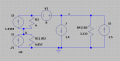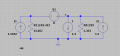# Transformation

#### Сергей999

Joined Jun 9, 2022
22
Have I correctly converted E1 and R1 into a current source?

#### Сергей999

Joined Jun 9, 2022
22
Is that right?

#### Irving

Joined Jan 30, 2016
3,548
I'm assuming you are trying to find the values for R3, E2, and J? You appear to be trying to apply Norton's theory to the circuit, which is a suitable approach (you could equally use Thevenin), but I'm not sure you've grasped it fully in terms of how you break it down.

To be sure I'm reading it right, you have these values:

R1 = 12Ω​
R2 = 16Ω​
R3 = unknown​
R4 = 5Ω​
R5 = 10Ω​
E1 = 9v​
E2 = unknown​
E3 =15v​
J = unknown​

So you have three unknowns; to solve that you need three simultaneous equations. However, by inspection we can see that:

I3 = I6​
and
E2 = R3 x I3

You can solve this circuit by developing the two Thevenin (or Norton, I'm not sure if one or the other is easier here) equivalents for the voltages at a and c, with R3 + R4||R5 as the load for Va and R3 + R1||R2 for Vc

Have another try...

#### Сергей999

Joined Jun 9, 2022
22
Converted, the current turned out to be 0.650A and should be 0.930A. E2=13V R3=9 Ohms What should I do?

#### Irving

Joined Jan 30, 2016
3,548

#### Сергей999

Joined Jun 9, 2022
22
We convert the voltage source E1 and R1 into a current source J1 and find a current of 9\12 = 0.75A. We convert the voltage source E3 and R5 into a current source J3 and find a current of 15\10 = 1.5A.
At the same time, we are dining R1,R2 and R4,R5.
Combine J3 and J, J4 = J3-J = 0.1A
Circuit current 0.75-0.1 = 0.65А
And it should be 0,93A

#### Irving

Joined Jan 30, 2016
3,548
We convert the voltage source E1 and R1 into a current source J1 and find a current of 9\12 = 0.75A. We convert the voltage source E3 and R5 into a current source J3 and find a current of 15\10 = 1.5A.
Agreed...

At the same time, we are dining R1,R2 and R4,R5.
Not sure what "dining" means, but agreed you parallel R1&R2 and R4&R5 so your equivalent circuit looks like:Combine J3 and J, J4 = J3-J = 0.1A
Circuit current 0.75-0.1 = 0.65А
And it should be 0,93A
Ok, this is where I don't understand your thinking - what was the original question? Were values for R3 and E2 given or not?

Is J4 the same as I4 (in the original schematic)? and is I4 given as 0.1A in the original.

What is 'circuit current'? Measured where?

#### Сергей999

Joined Jun 9, 2022
22
The translator translates this way into English.
E2 = 13B and R2 = 9 Om and J = 1.4 A were originally known.
0,65A we found.
And 0,93A was measured in LTspice
So I ask why the discrepancies between the calculated and the measured?

#### Сергей999

Joined Jun 9, 2022
22
Minimize the circuit by equivalent generators.
30 percent of the error, this is too much, means the calculation is not correct.

#### Irving

Joined Jan 30, 2016
3,548
Without seeing your experimental setup I can't comment, but I'd trust the simulation results on a simple DC analysis like this. If the experimental results don't match then most likely the physical circuit doesn't reflect the theoretical one. Are all currents and voltages in the right direction? How were you supplying J, E1, E2 and E3 and were they truely floating with respect to each other?

#### Сергей999

Joined Jun 9, 2022
22
Yeah, right.
The theoretical calculation is most likely not correct.

#### Irving

Joined Jan 30, 2016
3,548
The theoretical calculation, done from 1st principles, is unlikely to be wrong nor is the simulation at this simple level. If it doesn't match the real-world experiment then there must be some significant and identifiable difference between the theoretical model and the real world configuration - they are clearly not identical.

A little investigation shows that if R4 is disconnected at one end the current in R3 is 663mA, or if R4 and R5 are swapped over its 667mA - I'd say that either scenario is much more likely than the theory being wrong!

#### Сергей999

Joined Jun 9, 2022
22
And how to remove the dependence of current on R3?
Can it be transformed too?

#### Irving

Joined Jan 30, 2016
3,548
And how to remove the dependence of current on R3?
Can it be transformed too?
I'm not sure I understand - why would you want to do that? Is this not just a theoretical exercise, what would be the purpose of that?

#### Сергей999

Joined Jun 9, 2022
22
Still, theoretically, I did not decide correctly.
LTspice gives a different current.

#### Irving

Joined Jan 30, 2016
3,548
The only way to remove R3 is to remove E2, since I(R3) = -I(E2) and R3 . I(R3) = V(E2).

Since they are in series we can move E2 next to R3 and replace with the Thevenin equivalent:
(V1 here is just being used as a current sensor, it plays no active role)Then we can combine I1 and I3 (by isolating that branch to give the open circuit voltage Voc = 18.142v, and then experimentally showing the equivalent Thevenin resistance Rth when Vout = Voc/2 is actually R1||R2+R3 by running a DC Sweep. Finally, the equivalent Thevenin current is given by Voc/Rth).But we can never completely remove the influence of R3 as it is intrinsic to the circuit. If you run these simulations you will see that despite the changes

I(V1) = -928mA and​
V(c) = 3.427V​

always, thus demonstrating the theories are correct...

#### Сергей999

Joined Jun 9, 2022
22
But my calculations don't work, my calculations give 650mA?

#### Irving

Joined Jan 30, 2016
3,548
Then you aren't doing them correctly. Looking back at your posts, the problem seems to stem from this statement...

At the same time, we are dining R1,R2 and R4,R5.
Combine J3 and J, J4 = J3-J = 0.1A
Circuit current 0.75-0.1 = 0.65А
And it should be 0,93A
Justify that statement... draw the diagram...